Next: Gas Pipe (Fanno) Up: Fluid Section Types: Gases Previous: Carbon Seal   Contents

### Gas Pipe

The gas pipe element is a pipe element with constant cross section (Figure 83) It is allowed to rotate about an axis at a constant rotational speed. In that case the distance from the center point of the entrance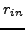and of the exit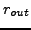to the rotational axis have to be specified.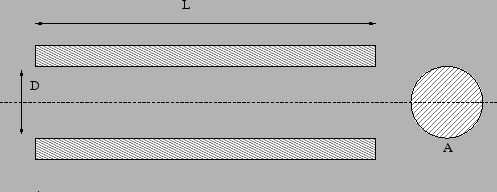The friction parameter is determined as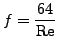(12)

for laminar flow ( Re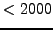) and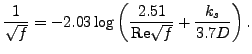(13)

for turbulent flow. Here,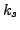is the diameter of the material grains at the surface of the pipe and Re is the Reynolds number defined by

 Re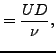(14)

where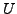is the liquid velocity and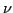is the kinematic viscosity. A gas pipe is described by the following parameters (to be specified in that order on the line beneath the *FLUID SECTION,TYPE=GAS PIPE ADIABATIC or *FLUID SECTION,TYPE=GAS PIPE ISOTHERMAL card):

• A: cross section of the pipe
• D: hydraulic diameter of the pipe defined as 4 times the area divided by the perimeter
• L: length of the pipe
•: grain diameter at the pipe surface
• form factor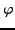of the cross section
• oil mass flow in the pipe (only if the OIL parameter is used to define the kind of oil in the *FLUID SECTION card)
• not used
• entry radius• exit radius• rotational speed

The default gas pipe is adiabatic, i.e. there is no heat exchange with the pipe. Alternatively, the user may specify that the pipe element is isothermal. This means that the static temperature does not change within the pipe. In that case the energy equation in one of the two corner nodes of the element is replaced by a isothermal condition.

The form factoris only used to modify the friction expression for non-circular cross sections in the laminar regime as follows: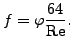(15)

Values forfor several cross sections can be found in . For a square cross section its value is 0.88, for a rectangle with a height to width ratio of 2 its value is 0.97.

Example files: gaspipe10, gaspipe8-cfd-massflow, gaspipe8-oil.Next: Gas Pipe (Fanno) Up: Fluid Section Types: Gases Previous: Carbon Seal   Contents
guido dhondt 2014-03-02